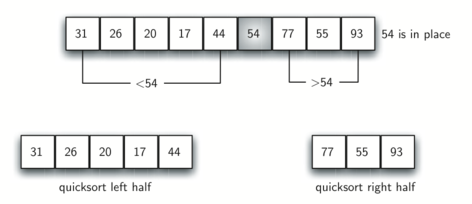# 5.12. The Quicksort¶

The quicksort uses divide and conquer to gain the same advantages as the merge sort, while not using additional storage. As a trade-off, however, it is possible that the list may not be divided in half. When this happens, we will see that performance is diminished.

A quicksort first selects a value, which is called the pivot value. Although there are many different ways to choose the pivot value, we will simply use the first item in the list. The role of the pivot value is to assist with splitting the list. The actual position where the pivot value belongs in the final sorted list, commonly called the split point, will be used to divide the list for subsequent calls to the quicksort.

Figure 12 shows that 54 will serve as our first pivot value. Since we have looked at this example a few times already, we know that 54 will eventually end up in the position currently holding 31. The partition process will happen next. It will find the split point and at the same time move other items to the appropriate side of the list, either less than or greater than the pivot value.

Partitioning begins by locating two position markers—let’s call them left_mark and right_mark—at the beginning and end of the remaining items in the list (positions 1 and 8 in Figure 13). The goal of the partition process is to move items that are on the wrong side with respect to the pivot value while also converging on the split point. Figure 13 shows this process as we locate the position of 54.

We begin by incrementing left_mark until we locate a value that is greater than the pivot value. We then decrement right_mark until we find a value that is less than the pivot value. At this point we have discovered two items that are out of place with respect to the eventual split point. For our example, this occurs at 93 and 20. Now we can exchange these two items and then repeat the process again.

At the point where right_mark becomes less than left_mark, we stop. The position of right_mark is now the split point. The pivot value can be exchanged with the contents of the split point and the pivot value is now in place (Figure 14). In addition, all the items to the left of the split point are less than the pivot value, and all the items to the right of the split point are greater than the pivot value. The list can now be divided at the split point and the quicksort can be invoked recursively on the two halves.Figure 14: Completing the Partition Process to Find the Split Point for 54¶

The quick_sort function shown in ActiveCode 1 invokes a recursive function, quick_sort_helper. quick_sort_helper begins with the same base case as the merge sort. If the length of the list is less than or equal to one, it is already sorted. If it is greater, then it can be partitioned and recursively sorted. The partition function implements the process described earlier.

To analyze the quick_sort function, note that for a list of length $$n$$, if the partition always occurs in the middle of the list, there will again be $$\log{n}$$ divisions. In order to find the split point, each of the $$n$$ items needs to be checked against the pivot value. The result is $$n\log{n}$$. In addition, there is no need for additional memory as in the merge sort process.

Unfortunately, in the worst case, the split points may not be in the middle and can be very skewed to the left or the right, leaving a very uneven division. In this case, sorting a list of $$n$$ items divides into sorting a list of 0 items and a list of $$n - 1$$ items. Then sorting a list of $$n - 1$$ divides into a list of size 0 and a list of size $$n - 2$$, and so on. The result is an $$O(n^{2})$$ sort with all of the overhead that recursion requires.

We mentioned earlier that there are different ways to choose the pivot value. In particular, we can attempt to alleviate some of the potential for an uneven division by using a technique called median of three. To choose the pivot value, we will consider the first, the middle, and the last element in the list. In our example, those are 54, 77, and 20. Now pick the median value, in our case 54, and use it for the pivot value (of course, that was the pivot value we used originally). The idea is that in the case where the first item in the list does not belong toward the middle of the list, the median of three will choose a better “middle” value. This will be particularly useful when the original list is somewhat sorted to begin with. We leave the implementation of this pivot value selection as an exercise.

Self Check